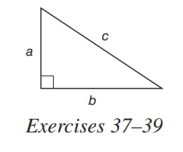# In Exercises 37-39, c 2 = a 2 + b 2 . In the right triangle, find c if a = 3 a n d b = 4 .### Elementary Geometry for College St...

6th Edition
Daniel C. Alexander + 1 other
Publisher: Cengage Learning
ISBN: 9781285195698

#### Solutions

Chapter
Section### Elementary Geometry for College St...

6th Edition
Daniel C. Alexander + 1 other
Publisher: Cengage Learning
ISBN: 9781285195698
Chapter A.5, Problem 37E
Textbook Problem
3 views

## In Exercises 37-39, c 2 = a 2 + b 2 .In the right triangle, find c if a = 3     a n d     b = 4 .To determine

To find:

To find c if a=3andb=4.

### Explanation of Solution

Consider the following equation,

c2=a2+b2.

The diagrammetic representation is given below,

Substitute the values of a=3andb=4 in the above equation to get the following,

c2=a2+b2c2=32+42c2

### Still sussing out bartleby?

Check out a sample textbook solution.

See a sample solution

#### The Solution to Your Study Problems

Bartleby provides explanations to thousands of textbook problems written by our experts, many with advanced degrees!

Get Started

Find more solutions based on key concepts
In Exercises 11-16, find the slope of the line that passes through each pair of points. 13. (2, 3) and (4, 8)

Applied Calculus for the Managerial, Life, and Social Sciences: A Brief Approach

If g(x) = 3 + x + ex, find g1(4).

Single Variable Calculus: Early Transcendentals

Finding an Indefinite Integral In Exercises 3-22, find the indefinite integral. dx14x2

Calculus: Early Transcendental Functions (MindTap Course List)

π does not exist

Study Guide for Stewart's Single Variable Calculus: Early Transcendentals, 8th

Find all real solutions of each equation. x2+1=7x+116

College Algebra (MindTap Course List)